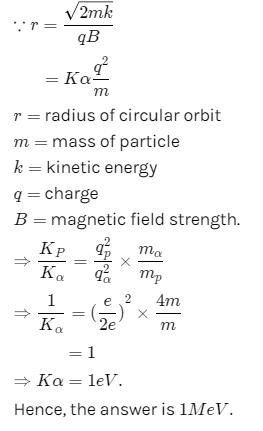# JEE(MAIN) Physics Mock Test - 8

## 30 Questions MCQ Test JEE Main Mock Test Series 2021 & Previous Year Papers | JEE(MAIN) Physics Mock Test - 8

Description
This mock test of JEE(MAIN) Physics Mock Test - 8 for JEE helps you for every JEE entrance exam. This contains 30 Multiple Choice Questions for JEE JEE(MAIN) Physics Mock Test - 8 (mcq) to study with solutions a complete question bank. The solved questions answers in this JEE(MAIN) Physics Mock Test - 8 quiz give you a good mix of easy questions and tough questions. JEE students definitely take this JEE(MAIN) Physics Mock Test - 8 exercise for a better result in the exam. You can find other JEE(MAIN) Physics Mock Test - 8 extra questions, long questions & short questions for JEE on EduRev as well by searching above.
QUESTION: 1

Solution:
QUESTION: 2

### The working of dynamo is based on principle of

Solution: The dynamo operates on the principle of the production of dynamically induced emf. Hence when ever flux is cut by the conductor, emf is poduced in it according to the law of electromagnetic induction.
QUESTION: 3

### A constant force acts on a body of mass 0.9 kg at rest for 10 s. If the body moves a distance of 250 m, the magnitude of the force is

Solution:
Here d= 250m
t= 10 seconds
initial velocity(u)= 0
using equation d= ut +1/2 at2, we will get
250 = 50 xa
a=5m/s2
force= mass x acceleration = 0.9ks x 5m/s2 = 4.5 newton
QUESTION: 4

Two bodies of mass 10 kg and 5 kg moving in concentric orbits of radii R and r such that their periods are the same. Then the ratio between their centripetal acceleration is

Solution: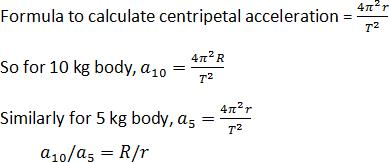QUESTION: 5
The directions of electric and magnetic fields in J.J. Thomson's experiment for the determination of e/m for an electron are
Solution: The specific charge of an electron can be determined when electron moves in both magnetic field and electric field which are mutually perpendicular to each other so that the net force on the electron is made zero. IN this situation the direction of motion of electron remains perpendicular to both electric and magnetic field.
QUESTION: 6

An electric dipole is placed along the X-axis at the origin O. A point P is at a distance of 20 cm from this origin such that OP makes an angle π/3 with the X axis. If electric field at P makes an angle θ with X-axis, the value of θ is

Solution: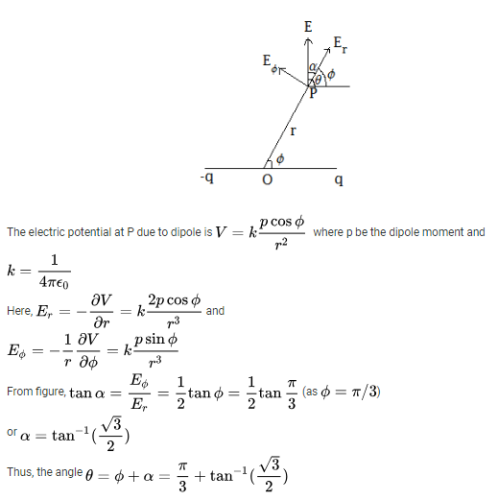QUESTION: 7
A glof ball of mass 0.05 kg placed on a tee, is struck by a golf club. The speed of the golf ball as it leaves the tee is 100 m/s, the time of contact between them is 0.02 s. If the force decreases to zero linearly with time, then the force at the beginning of the contact is
Solution:
QUESTION: 8

A semi circle arc of radius 'a' is charged uniformly and the charge per unit length is λ. The electric field at its centre is

Solution:
QUESTION: 9

The figure shows the symbol of a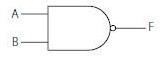Solution:
QUESTION: 10
A particle is moving in a uniform magnetic field then
Solution:
QUESTION: 11
A liquid flows through a pipe of non-uniform cross-section. If A₁ and A₂ are the cross-sectional areas of the pipe at two points, the ratio of velocities of the liquid at these points will be
Solution:
QUESTION: 12

Which of the following equations is definitely wrong?

Solution:
QUESTION: 13
In neutron discovery experiment, Berillium element is bombarded by
Solution:
QUESTION: 14
In the following question, a Statement of Assertion (A) is given followed by a corresponding Reason (R) just below it. Read the Statements carefully and mark the correct answer-
Assertion(A): A particle move from A (X1,Y1,Z1) to B (X2,Y2,Z2), displacement is given as (X2-X1)i, (Y2-Y1)j, (Z2-Z1)k.
Reason (R):Displacement can be positive, negative or zero.
Solution:
QUESTION: 15
In the following question, a Statement of Assertion (A) is given followed by a corresponding Reason (R) just below it. Read the Statements carefully and mark the correct answer-
Assertion(A): The density of air at the top of the troposphere is about 10 times the density near the earth's surface.
Reason(R): The atmosphere between the heights of 12 km and 50 km is called troposphere.
Solution:
QUESTION: 16
For a gas, if the ratio of specific heats at constant pressure and volume is γ, then the value of degree of freedom is
Solution: Cp=(f/2+1)RT
Cv= (f/2)RT
Taking ratio we get
Cp/Cv=¥=(f/2+1)/(f/2)
¥=(f+2)/f
¥=1+2/f
¥-1=2/f
f=2/(¥-1)
Hope it helps
QUESTION: 17

A 2 kg mass is rotating on a circular path of radius 0.8 m with angular velocity of 44 rad-s-1. If radius of the path becomes 1 m, then value of angular velocity will be

Solution: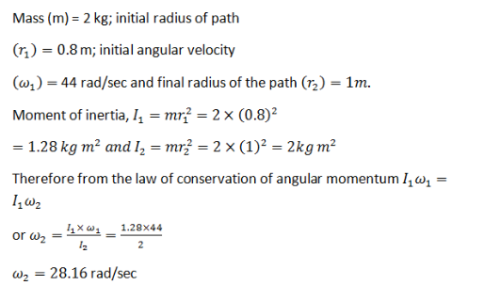QUESTION: 18

Which is not a unit of electric field

Solution:
QUESTION: 19
Ultrasonic waves are those waves
Solution:
QUESTION: 20

In a mixture of H−He+ gas (He+ is singly ionized He atom) , H atoms and He+ ions are excited to their respective first excited states. Subesquently, H atoms transfer their total excitation energy to He+ ions (by collisions). Assume that the Bohr model of atom is exactly vaild.

Q. The quantum number n of the state finally populated in He+ ions is

Solution:
QUESTION: 21

In a mixture of H−He+ gas (He+ is singly ionized He atom) , H atoms and He+ ions are excited to their respective first excited states. Subesquently, H atoms transfer their total excitation energy to He+ ions (by collisions). Assume that the Bohr model of atom is exactly vaild.

Q. The wavelength of light emitted in the visible region by He+  ions after collisions with H atoms is

Solution:
QUESTION: 22

In an Young's double slit experiment setup, as shown in the figure, the light source executes simple harmonic motion between P and Q according to the equation x=Asinωt, s being the mean position. Assume d→O and A≪L. ω is small enough to neglect Doppler effect. If the source were stationary at s, intensity at O would be I0.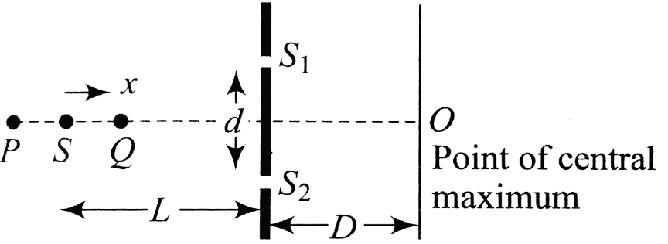Q. When the source comes towards the point Q,

Solution:
QUESTION: 23

In an Young's double slit experiment setup, as shown in the figure, the light source executes simple harmonic motion between P and Q according to the equation x=Asinωt, s being the mean position. Assume d→O and A≪L. ω is small enough to neglect Doppler effect. If the source were stationary at s, intensity at O would be I0.Q. The fringe width β can be expressed as

Solution:
QUESTION: 24

In an Young's double slit experiment setup, as shown in the figure, the light source executes simple harmonic motion between P and Q according to the equation x=Asinωt, s being the mean position. Assume d→O and A≪L. ω is small enough to neglect Doppler effect. If the source were stationary at s, intensity at O would be I0.Q. The fractional change in intensity of the central maximum as function of time is

Solution:
QUESTION: 25

A 5.0 μ F capacitor is charged to a potential difference of 800 V and discharged through a conductor. The energy given to the conductor during the discharge is

Solution:
QUESTION: 26

A charge is moving in a uniform magnetic field in a circular path of radius R. If the energy of the particle is doubled, the new radius is

Solution:
QUESTION: 27

A uniform rod of length l is freely pivoted at one end. It is initially held horizontally and then released from rest. The angular velocity at the instant when the rod is vertical is

Solution:
QUESTION: 28

Two identical thin conducting spherical shells of radius R carry equal negative charges of magnitude Q sprayed uniformly on their surfaces. The distance AB between their centres is 4R. The electric field at a point P(fig.) at distance R/2 from the centre A of the first sphere is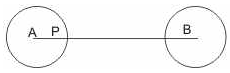Solution:
QUESTION: 29

An electric iron draws 5 amp, a TV set draws 3 amp and a refrigerator draws 2 amp from a 220 volt main line. The three appliances are connected in parallel. If all the three are operating at the same time, the fuse used may be of

Solution:
QUESTION: 30

A proton of mass m and charge + e is moving in a circular orbit of magnetic field with energy 1 Me V. What should be the energy of α-particle (mass 4 m and charge + 2 e) so that it can revolve in the path of same radius as that of proton.

Solution: